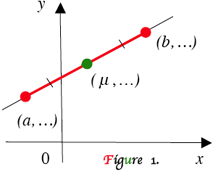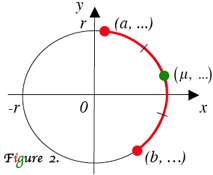Math Central - mathcentral.uregina.caAn Arc Midpoint Computation Lesson

Gregory V. Akulov, teacher,
Luther College High School, Regina

and
Oleksandr (Alex) G. Akulov, student,
University of Waterloo

February 25, 2011Resource RoomThe well-known midpoint formula tells that, if the straight line’s segment has the ends at $x = a, b,$ and midpoint at $x = \mu$, then $2 \mu = a + b \mbox{ or } \mu = \large \frac{a + b}{2}.$ It also tells that the same relationship holds for y-values. Circular analogue of midpoint formula is a new topic.# Arc Midpoint Computation

Let origin-centered arc of radius $r$ (see Figure 2) have the ends at $x = a, b,$ and midpoint at $x = \mu.$ Then

$2 \small \mu = \pm \sqrt{(r + a)(r + b)} \pm \sqrt{(r - a)(r - b)}\; ,$

where the first radical gets “−” iff1 the arc has a negative x-intercept, and
the second radical gets “+” iff  the arc  has a positive x-intercept.

### The same relationship holds for y-values.

#### Examples

A .  Origin-centered arc of radius 50,   located as shown at Figure 2, has the ends at $x = 14$ and $x = 25$. Find x-coordinate of its midpoint.
B . An arc of radius 40 is centered at origin. It starts and ends a $x = \;‒24$  and $x = 9$. What is x-coordinate of arc’s midpoint, if the arc is located in quadrants I and II?
C . An arc has center at $(0, 0)$ and radius 82. It starts at $y = 18$ in quadrant II, passes through quadrants III and ends in quadrant IV at $y = \;‒1$. What is y-value of arc’s midpoint?#### Solutions

A . $r = 50, a = 14, b = 25,$ both radicals go with “+”, and $20 \sqrt{3} + 15$ is the answer;
B
. $r = 40, a = ‒24, b = 9,$ first radical goes with “+”, second radical goes with “−”,  and $14 - 4 \sqrt{31}$ is the answer;
C .
$r = 82, a = 18, b = \;‒1,$ both radicals go with “−”, and $-45 - 4 \sqrt{83}$ is the answer.

Arc midpoint computation was suggested by first attempt shown in . Details of proof involved identities received from .

References

1. Oleksandr (Alex) G. Akulov (2009). Dot product finds arc midpoint. Math News, U of W. Volume 111, Issue 6, p. 5.
2. Gregory V. Akulov (2010). The slope of the angle bisector relationship in applied and theoretical problems. Vinculum, SMTS. Volume 2, Number 1, p. 49.
______________________
1The word iff first appeared in 1950s as a shortening of conjunction if and only if.

Copyright © January, 2011 by Gregory V. Akulov,  Oleksandr (Alex) G. Akulov

# Arc Midpoint Calculator 1

Oleksandr G. Akulov, MM in OR, BMath, Vancouver, British Columbia
Gregory V. Akulov, teacher, Luther College High School, Regina, Saskatchewan

Cartesian x-values of the endpoints, a, b, and the centre, c,

a =       b =       c =

If the arc has a point with x-value cr, enter  −1, otherwise 1
If the arc has a point with x-value c + r, enter 1, otherwise  −1
μ =

Irrelevant output indicates inaccurate input.

To calculate the y-value assume y instead of x in the above.

Copyright © May 19, 2016 by Oleksandr G. Akulov, Gregory V. Akulov
 Math Central is supported by the University of Regina and the Pacific Institute for the Mathematical Sciences.about math central :: site map :: links :: notre site français